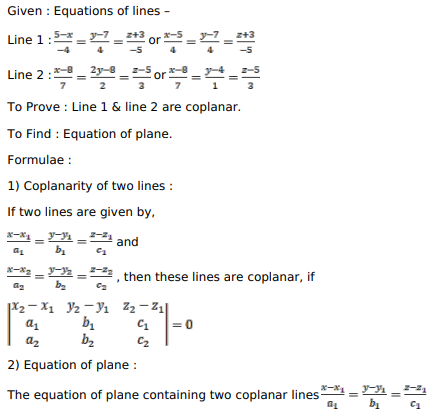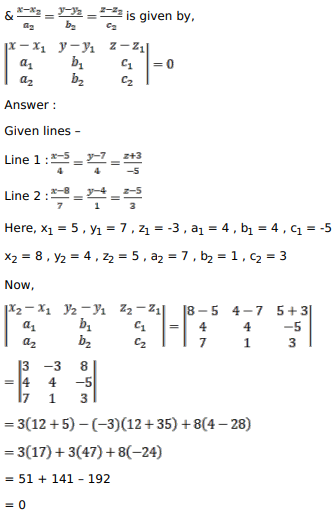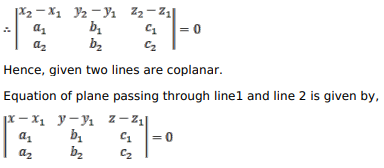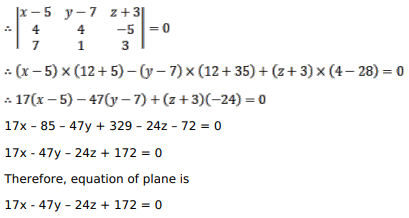# Solve this following

Question:

Show that the lines $\frac{5-x}{-4}=\frac{y-7}{4}=\frac{z-3}{-5}$ and $\frac{x-8}{7}=\frac{2 y-8}{2}=\frac{z-5}{3}$ are coplanar. Find the equation of the plane containing these lines.

Solution: